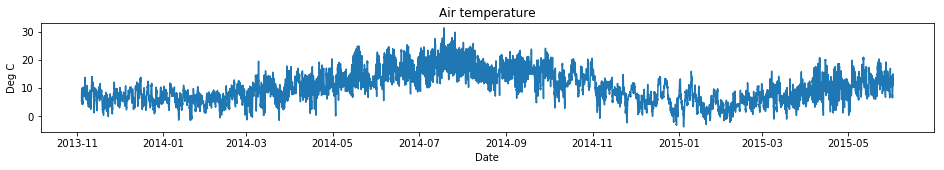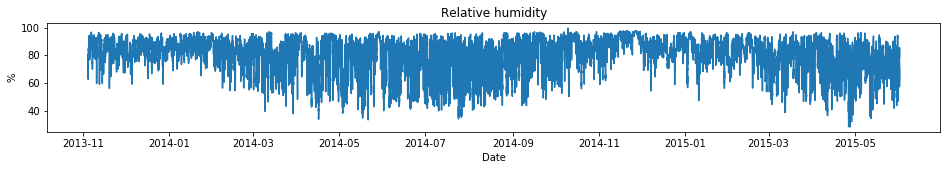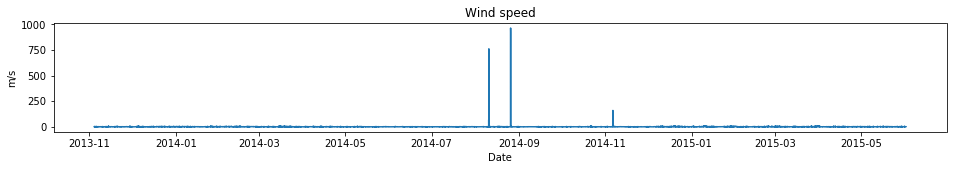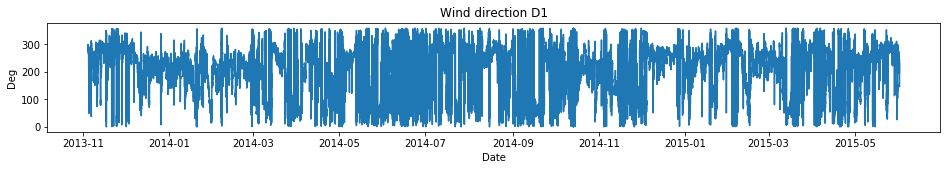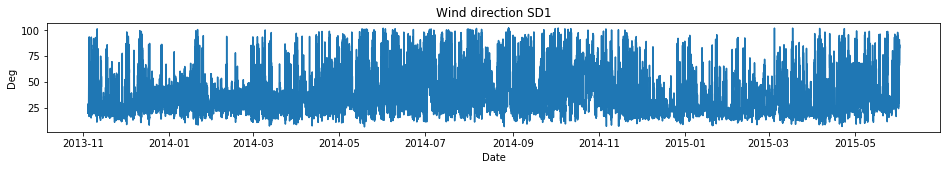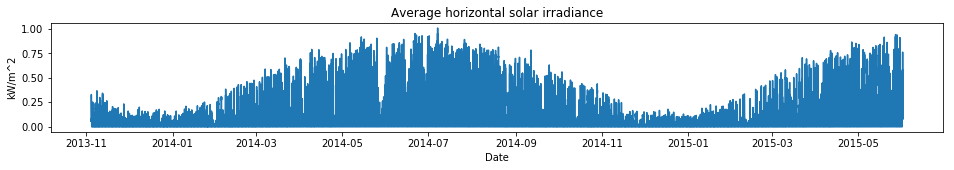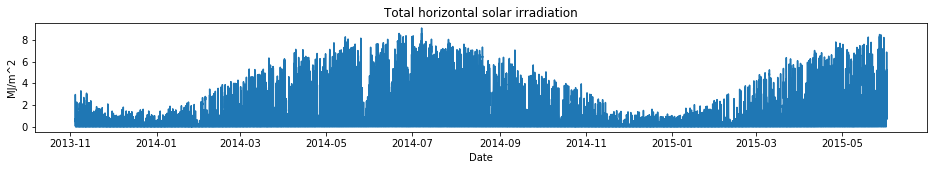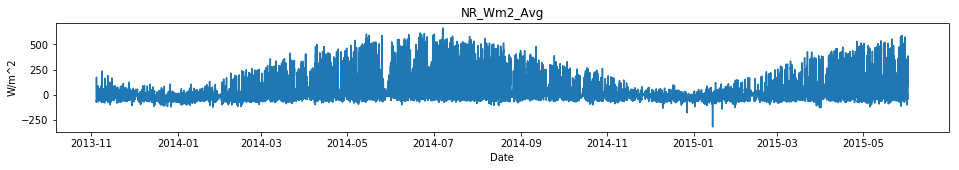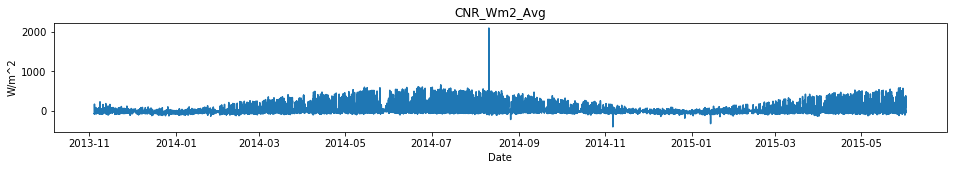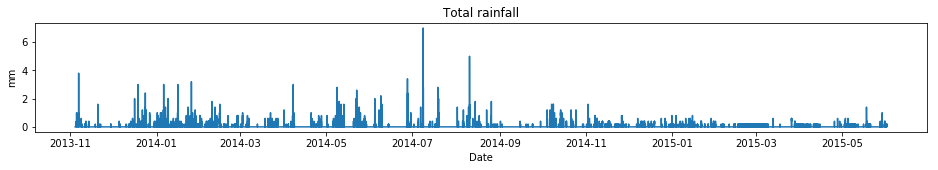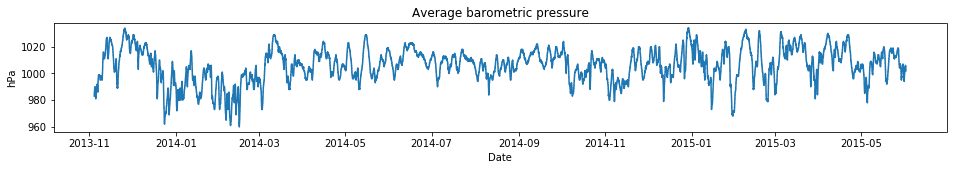## How do I plot the climate data?¶

By Steven Firth, s.k.firth@lboro.ac.uk, Loughborough University, UK

The climate data in the REFIT dataset can be plotted by:

1. Importing the relevant python packages and set up matplotlib for plotting
2. Reading the 'REFIT_BUILDING_SURVEY.xml' file into memory
3. Reading the 'REFIT_TIME_SERIES_VALUES.csv' file into memory
4. Looping through each climate TimeSeriesVariable and plotting the TimeSeriesValues

This notepook is developed using the 'jupyter' software and Python implementation in the Anaconda platform.

Step 1: Importing the relevant python packages and set up matplotlib for plotting

In :
from lxml import objectify
import pandas as pd
import matplotlib.pyplot as plt
%matplotlib inline


Step 2: Reading the 'REFIT_BUILDING_SURVEY.xml' file into memory

This loads the xml file into the lxml objectify data structure. 'root' can then be used to access the building survey data.

In :
path=r'REFIT_BUILDING_SURVEY.xml'
tree = objectify.parse(path)
root = tree.getroot()
NS={'a':'http://www.refitsmarthomes.org'}


Step 3: Reading the 'REFIT_TIME_SERIES_VALUES.csv' file into memory

This load the time series measurements into a pandas dataframe object named 'csv'. The 'csv' dataframe has:

• index column: the 'TimeSeriesVariable/@id' values (string)
• column 0: the 'dateTime' values (datetime64[ns])
• column 1: the 'data' values (float64)
In :
path=r'REFIT_TIME_SERIES_VALUES.csv'


Step 4: Looping through each climate TimeSeriesVariable and plotting the TimeSeriesValues

This uses an xpath expression to find the TimeSeriesVariable elements that are children of the Climate element in the XML file. By looping through these elements, the TimeSeriesValues are plotted on a separate graph for each variable.

Note - the plots show that there appear to be some error values in the data, for example the high values seen in the 'wind speed' variable. This suggests that the data should be checked and may need to be cleaned before carrying out any analysis.

In :
elements=root.xpath('./a:Stock/a:Climate/a:Sensor/a:TimeSeriesVariable', namespaces=NS)
for e in elements:
id = e.get('id')
variableType=e.get('variableType')
units=e.get('units')
fig, ax = plt.subplots(figsize=(16,2))
ax.set_title(variableType)
ax.set_xlabel('Date')
ax.set_ylabel(units)
ax.plot(csv.loc[id]['dateTime'],csv.loc[id]['data'])
plt.show()